College Physics : Energy Quantization

Example Questions

Example Question #1 : Quantum And Nuclear Physics

A laser outputs light of a single color with photon energy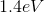. A nonlinear crystal, which halves the wavelength of light, is placed in the path of the laser beam. What is the energy of the photons exiting the crystal?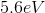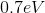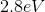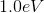Explanation:

Halving the wavelength is equivalent to doubling the frequency since for a light wave the wavelength and frequency are related by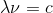, whereis the speed of light. The energy of a photon is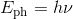, whereis Planck's constant, so the photon energy is doubled.

Example Question #1 : Energy Quantization

A monochromatic light source is incident on a neutral density filter which decreases the light source's intensity by a factor of 3 but does not change its color. What happens to the energy of a photon in the less intense beam?

The photon energy decreases by a factor of 3.

The photon energy decreases only slightly.

The photon energy decreases by a factor of 9.

The photon energy increases by a factor of 3.

None of these